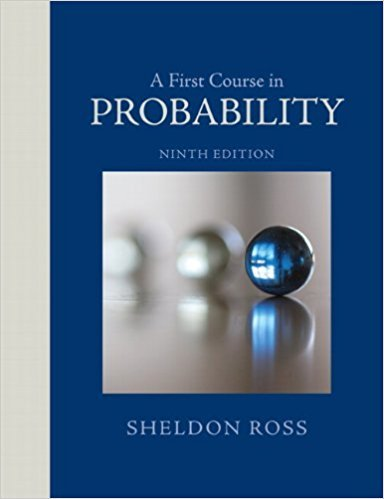×
Get Full Access to A First Course In Probability - 9 Edition - Chapter 3 - Problem 28te
Get Full Access to A First Course In Probability - 9 Edition - Chapter 3 - Problem 28te

×

# Prove or give a counterexample. If E1 and E2 areISBN: 9780321794772 63

## Solution for problem 28TE Chapter 3

A First Course in Probability | 9th Edition

• Textbook Solutions
• 2901 Step-by-step solutions solved by professors and subject experts
• Get 24/7 help from StudySoup virtual teaching assistantsA First Course in Probability | 9th Edition

4 5 1 283 Reviews
29
2
Problem 28TE

Problem 28TE

Prove or give a counterexample. If E1 and E2 are independent, then they are conditionally independent given F.

Step-by-Step Solution:

Solution:

Step 1 of 1:

Two eventsandare conditionally (given F) independent .

We have to prove or to give a counterexample.

if Q(= Q(Q(Step 2 of 1

##### ISBN: 9780321794772

The full step-by-step solution to problem: 28TE from chapter: 3 was answered by , our top Statistics solution expert on 08/11/17, 08:57AM. This full solution covers the following key subjects: independent, given, give, Counterexample, conditionally. This expansive textbook survival guide covers 4 chapters, and 469 solutions. Since the solution to 28TE from 3 chapter was answered, more than 1206 students have viewed the full step-by-step answer. This textbook survival guide was created for the textbook: A First Course in Probability , edition: 9. A First Course in Probability was written by and is associated to the ISBN: 9780321794772. The answer to “Prove or give a counterexample. If E1 and E2 are independent, then they are conditionally independent given F.” is broken down into a number of easy to follow steps, and 18 words.

Unlock Textbook Solution